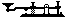## International Morse Code

#### Text Input -- Morse Code Output

Enter text characters into the upper text area under the "Input" heading. Select the "Translate" button to see the Morse code equivalent of the text. This will be displayed in the lower text area under the "Translation" heading. Select the "Play" button to start sounding the Morse code and viewing the code's equivalent text. Use the flip down menu to select the desired code speed.

#### Morse Code Input -- Text Output

Enter Morse code character symbols into the upper text area under the "Input" heading. Use a period (.) for the dot (dit) and a hyphen (-) for the dash (dah). Put a space between letters and divide words using a "/" or "|". Select the "Translate" button to see the text equivalent of the Morse code. This will be displayed in the lower text area under the "Translation" heading. Select the "Play" button to start sounding the Morse code and viewing its text equivalent. If a character cannot be properly translated, a "*" will be displayed.

#### Notes

The following character groups are the ones that I found to be very helpful when I was studying to become a ham at the age of 12. The ham radio bug had bitten and I tried just about everything to simplify the code learning process. I could hardly wait to pass the test and get my novice license. These are some character symbol groups that can be used to simplify rhythm recognition practice. Be sure to listen to the rhythm of these characters as they are studied. The recommended character groups are as follows:

 Character Symbol Groups All Dots All Dashes Opposites E = dit I = di-dit S = di-di-dit H = di-di-di-dit T = dah M = dah-dah O = dah-dah-dah A = di-dah B = dah-di-di-dit D = dah-di-dit E = dit F = di-di-dah-dit G = dah-dah-dit I = di-dit K = dah-di-dah L = di-dah-di-dit O = dah-dah-dah P = di-dah-dah-dit N = dah-dit J = di-dah-dah-dah W = di-dah-dah T = dah Q = dah-dah-di-dah U = di-di-dah M = dah-dah R = di-dah-dit Y = dah-di-dah-dah S = di-di-dit X = dah-di-di-dah

"Have KeyWill Send"